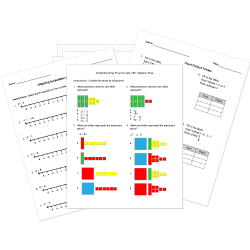Looking for Algebra worksheets?
Check out our pre-made Algebra worksheets!
 Tweet##### Browse Questions
• Arts (231)
• English Language Arts (1767)
• English as a Second Language ESL (785)
• Health and Medicine (449)
• Life Skills (702)
• Math (634)

• ### Vectors

• #### Trigonometry

• Physical Education (235)
• Science (1641)
• Social Studies (1230)
• Study Skills and Strategies (32)
• Technology (122)
• Vocational Education (197)

You can create printable tests and worksheets from these Grade 12 Function and Algebra Concepts questions! Select one or more questions using the checkboxes above each question. Then click the add selected questions to a test button before moving to another page.

Previous Next
Grade 12 Complex Numbers CCSS: HSN-CN.C.8
Factor. $x^2+12$
1. $2sqrt3i$
2. $(x-4i)(x+4i)$
3. $+-sqrt12$
4. $(x+2sqrt3i)(x-2sqrt3i)$
Grade 12 Polynomials and Rational Expressions CCSS: HSA-APR.C.5
How can one determine the coefficients of the Binomial Theorem expansion for $(x+y)^n$, where $n$ is an integer? There may be more than one correct answer.
1. Pascal's Triangle
2. Binomial Permutation
3. $({:(n),(k):}), \ k=0,...,n$
4. $(n!)/(k!(n-k)!), \ k=0,...,n$
If the matrix $[[27,9,12],[3,0,6],[18,21,3]]$ is multiplied by the scalar $1/3$, what is the result?
1. $[[9,3,4],[1,0,2],[6,7,1]]$
2. $[[30,12,14],[6,3,9],[21,24,6]]$
3. $[[27,9,12],[3,0,6],[18,21,3]]$
4. $[[9,1,6],[3,0,7],[4,2,1]]$
Grade 12 Polynomials and Rational Expressions CCSS: HSN-CN.C.9
If A is a given square matrix, and it is known that there exists a matrix B such that $AB=1$, which of the following would be the most efficient ways to find the matrix B?
1. Find the inverse of A. This is the matrix B.
2. Find the transpose of A. This is the matrix B.
3. Create a matrix B whose elements are variables. Then, perform matrix multiplication with the matrix A, setting each resulting entry equal to one. Solve this system of equations, which will give the elements of matrix B.
4. Multiply both sides of the equation, on the left, by slight variations of the matrix A. When one of these matrices, multiplied by A, becomes the identity matrix, this is the matrix B.
Grade 12 Polynomials and Rational Expressions CCSS: HSA-APR.D.6
What is the remainder of $x^2+6x-2$ divided by $x-5$?
1. $-7$
2. $x-53$
3. $53$
4. $-2$
If Matrix A = $[(-3,1),(-2,4),(5,-1)]$ and Matrix B = $[(4,-3),(0,-2),(-2,4)]$, then what is 3A - 2B?
1. $[(-1,-3),(-6,8),(11,5)]$
2. $[(-1,9),(-6,8),(11,5)]$
3. $[(-1,9),(-6,8),(11,-11)]$
4. $[(-17,9),(-6,16),(19,-11)]$
Two vectors with the same direction are equal.
1. They are always equal.
2. They are never equal.
3. It depends on their magnitude.
4. It depends on their end point.
Two vectors with the same magnitude are equal.
1. They are always equal.
2. They are never equal.
3. It depends on their direction.
4. It depends on their initial point.
James needs to show that for matrix $A = [[5,-2,1],[3,3,2],[-6,3,-1]]$, there is no matrix $B, B!=I$, such that $AB = I$, where $I$ is the 3-by-3 identity matrix. How can he do this?
1. Try at least 3 matrices, and if none of them multiplied by $A$ equal the identity matrix, then it is not possible.
2. Find the inverse of $A$, and then show that since this matrix is unique, there cannot exist another matrix $B$ such that $AB = I$.
3. Subtract by the additive inverse on both sides, and then factor the left hand side of the equation. This implies that if $B=I$ the equation equals the zero matrix, which it can't.
4. Show that the determinant of $A$ is zero, which means that it does not have a multiplicative inverse.
Find the difference. $[[2,0],[-4,1],[8,12],[0,-3],[1,1]] - [[-3,5],[6,10],[3,12],[-4,4],[8,7]]$
1. $[[5,5],[-2,-9],[-5,-24],[-4,1],[-7,-6]]$
2. $[[5,-5],[-10,-9],[5,0],[4,-7],[-7,-6]]$
3. $[[-1,5],[2,11],[11,24],[-4,1],[9,8]]$
4. $[[-5,-5],[-2,9],[5,24],[-4,-7],[7,6]]$
Add. $[[4,9,-6],[-3,0,8],[2,2,5]] + [[-10, 2,6],[5,-7,-8],[2,12,-5]]$
1. $[[-6,11,0],[2,-7,0],[4,14,0]]$
2. $[[14,11,12],[8,-7,16],[4,14,10]]$
3. $[[-14, 7,-12],[-8,7,16],[0,-10,10]]$
4. $[[-6,11],[2,-7],[4,14]]$